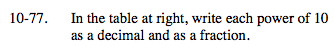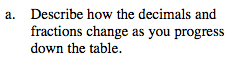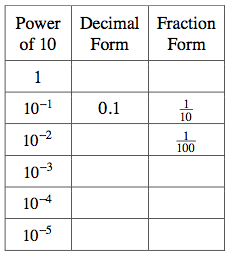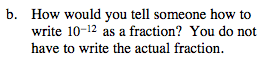### Home > MC2 > Chapter 10 > Lesson 10.1.6 > Problem10-77

10-77.10−2 = 0.01
10−3 = 0.001

Do you notice any patterns? Try to fill in the rest of the table on your own.Notice that the decimal point moves to the left one spot as the value of the exponent decreases, while the denominator in the fraction form increases by a multiple of 10.From the table, you see that the magnitude of the exponent corresponds to the number of zeros in the denominator of the fraction form of the number.

Therefore the fraction form of this number would have 1 over a denominator with 12 zeros.